# drylin® ZLW - Toothed belt axis - Drive torque and load

### Drive torque and fill weight of the ZLW-1040/1080

#### Required drive torque*Vertical orientation – ZLW-1040/1080, Version basic 02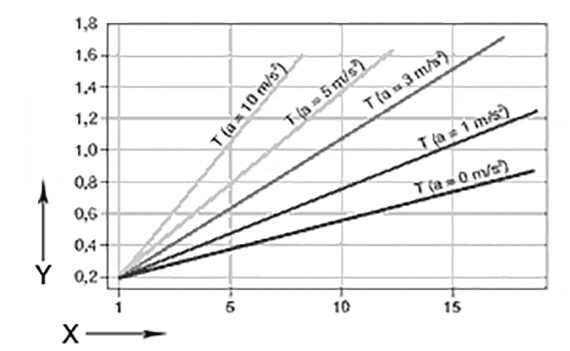X = Load capacity[kg]
Y = drive torque [Nm]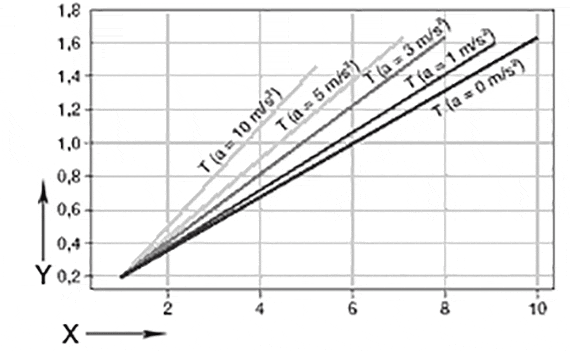X = Load capacity[kg]
Y = drive torque [Nm]

#### Required drive torque*Vertical orientation – ZLW-1040/1080, Version standard 02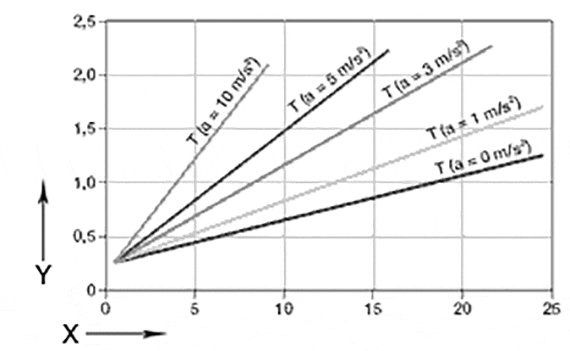X = Load capacity[kg]
Y = drive torque [Nm]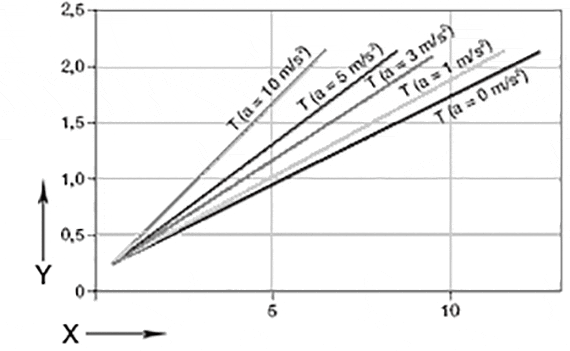X = Load capacity[kg]
Y = drive torque [Nm]

* Supposition: The moving mass is located in a circumscribed circle with a max. R = 100 mm to the middle of the rail, max. permissible torque, version 01: 1.3 Nm, a= 0 m/s² ; version 02: 2.4 Nm, a= 0 m/s²; constant drive without nominal value acceleration.

#### Sag between unsupported end blocksZLW-1040/1080, Version basic 02 and standard 02.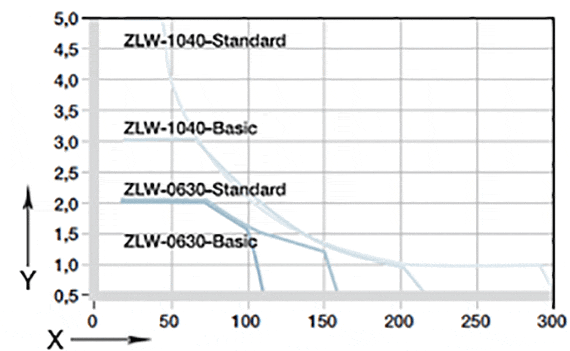X = load of the carriage [N]
Y = speed of the carriage [m/s]

The diagram accounts for the sum of all forces active on the carriage. ED = On-time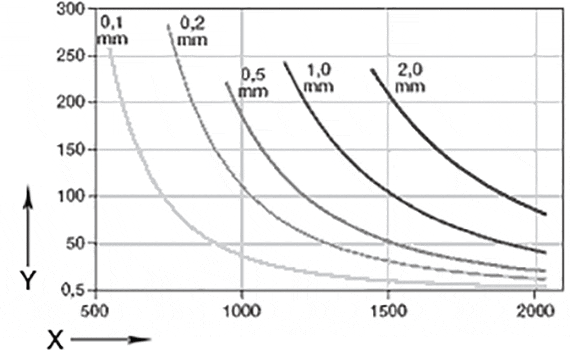X = Support length [mm]

Sag permissible up to maximum 2 mm .

### Drive torque and load of the ZLW-0630

#### Required drive torque*Installation position vertical - ZLW-0630, basic version 02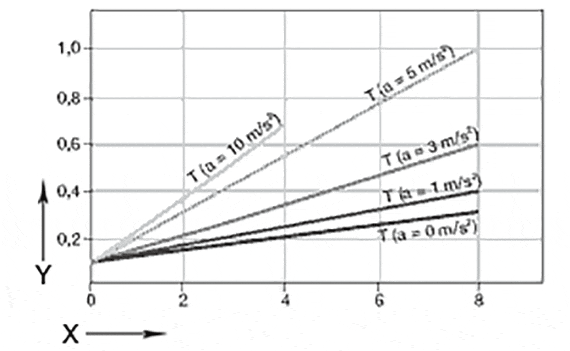X = Load capacity[kg]
Y = drive torque [Nm]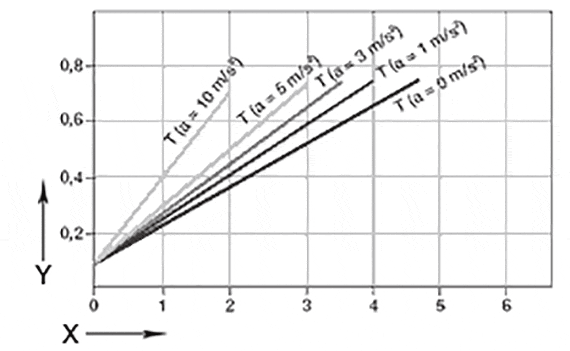X = Load capacity[kg]
Y = drive torque [Nm]

#### Required drive torque*Installation position vertical - ZLW-0630, standard version 02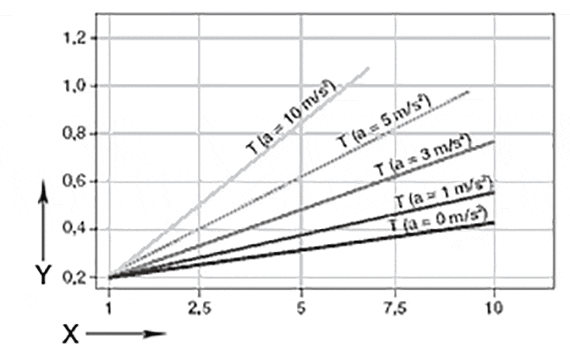X = Load capacity[kg]
Y = drive torque [Nm]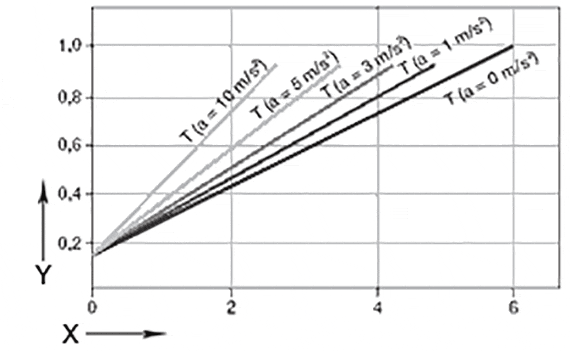X = Load capacity[kg]
Y = drive torque [Nm]

* Supposition: The mass to be moved is in a circumscribed circle with a max. R = 100 mm to the centre of the guide rail, max. permitted torque, version 01: 1.3 Nm, a= 0 m/s² ; version 02: 2.4 Nm, a= 0 m/s²; constant drive without nominal value acceleration.

#### Bending under load based on the width between supportsZLW-0630, basic version 02 and standard 02X = load of the carriage [N]
Y = speed of the carriage [m/s]

The diagram accounts for the sum of all forces active on the carriage. ED = On-time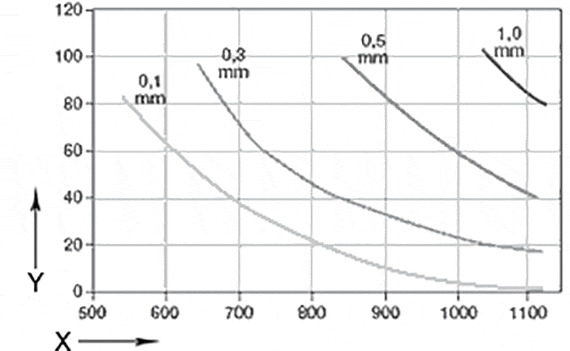X = Support lenght [mm]

Sag permissible up to maximum 2 mm .

### Drive torque and fill weight of the ZLW-1660

#### Required drive torque*Vertical orientation – ZLW-1660, Version standard 02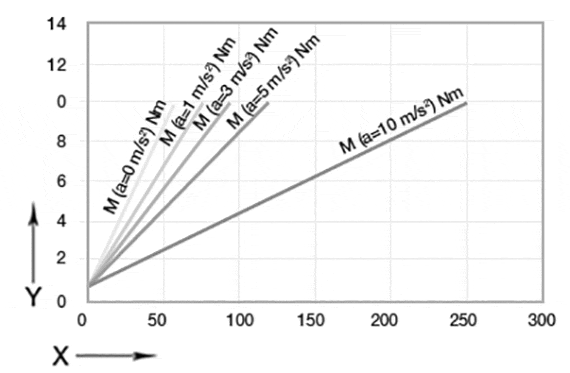X = payload [kg]
Y = torque [Nm]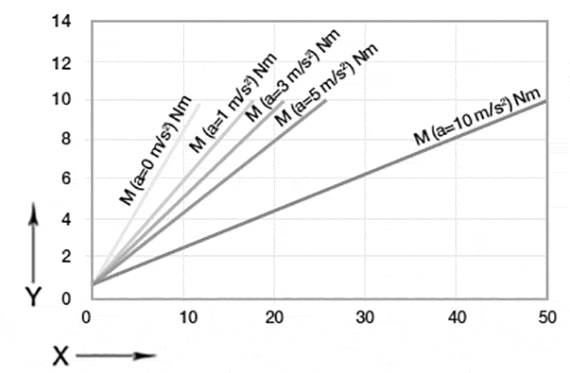X = payload [kg]
Y = torque [Nm]

*Assumption: the moving mass is located in a circumscribed circle with a Max. R = 100 mm to the middle of the guiding rail, max. permissible torque Version 01: 1.3 Nm, a = 0 m/s²; Version 02: 2.4 Nm, a = 0 m/s²; constant drive without nominal acceleration value

#### Maximum load,horizontal orientation; the diagram accounts for the sum of all forces active on the carriage.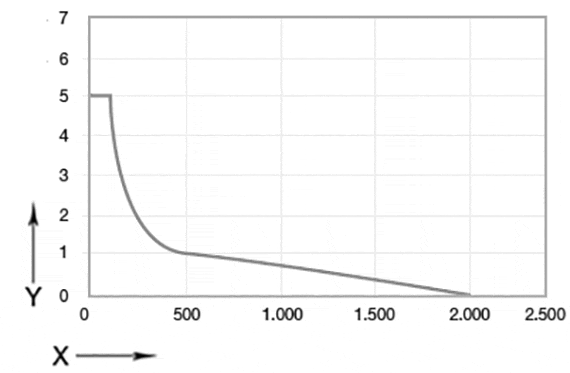X = Carriage load[N]
Y = Carriage speed [m/s]